# Math in Focus Grade 2 Chapter 6 Practice 3 Answer Key Multiplying 5: Skip-counting

Go through the Math in Focus Grade 2 Workbook Answer Key Chapter 6 Practice 3 Multiplying 5: Skip-counting to finish your assignments.

## Math in Focus Grade 2 Chapter 6 Practice 3 Answer Key Multiplying 5: Skip-counting

Jon is skip-counting by 5s. He shades each number he counts on a hundreds chart. He misses some numbers. Circle the numbers he misses.

Question 1.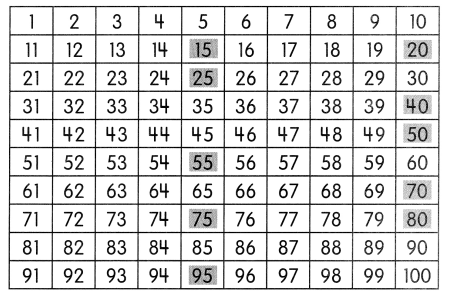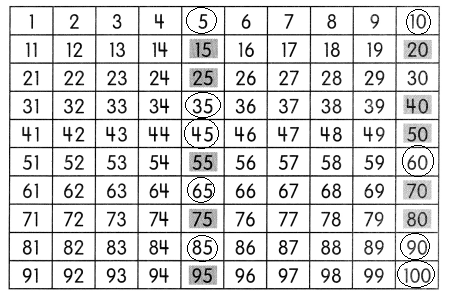Find the missing numbers.

3 groups of 5 = 3 × 5
= 15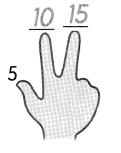Question 2.
4 groups of 5 = ___ × 5
= _______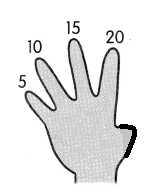4 groups of 5 =4 × 5
= 20

Question 3.
5 groups of 5 = ___ × ____
= _____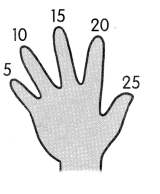5 groups of 5 = 5 ×  5
= 25

Question 4.
7 groups of 5 = ___ × ____
= _____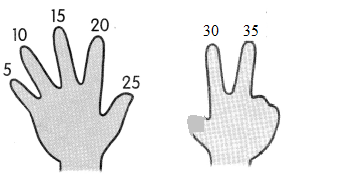7 groups of 5 = 7 × 5
= 35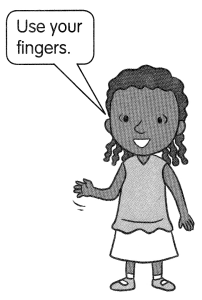Question 5.
8 groups of 5 = ___ × ____
= ______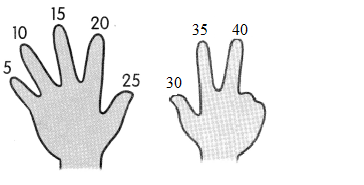8 groups of 5 = 8 × 5
= 40

Question 6.
9 groups of 5 = ___ × _______
= ________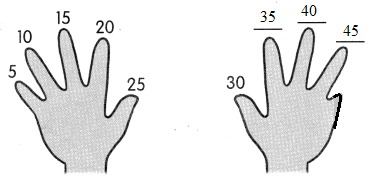9 groups of 5 = 9 × 5
= 45

Count by 5s.
Then fill ¡n the blanks.

Question 7.
5, 10, 15, 20, 25, ____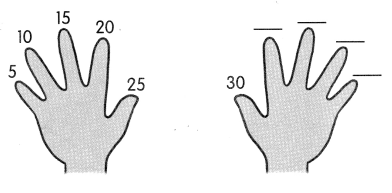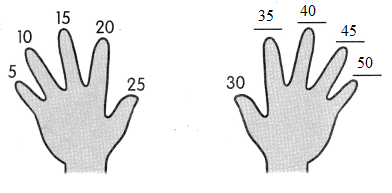5, 10, 15, 20, 25, 30,35,40,45,50

Question 8.
20, 25, __, ___, ___, ___, ____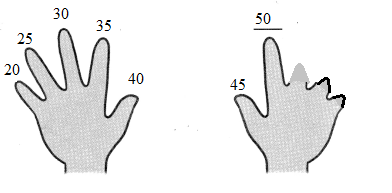20,25,30,35,40,45,50

Count by 5s.

Then fill in the blanks.

Question 9.
20, ____, 30, ___, 40, 45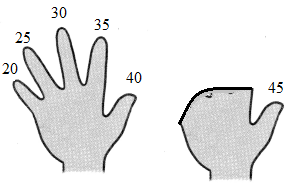20,25,30,35,40,45

Question 10.
____, ____, 25, 30, 35, ___, ___, ____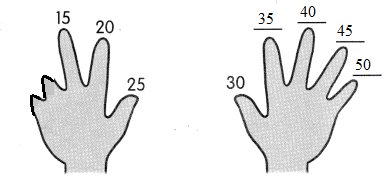15,20,25,30,35,40,45,50

Fill in the blanks.

Question 11.
3 × 5 = ___
3 × 5 = 15
3 groups of five is equal to 15

Question 12.
2 × 5 = ____
2 × 5 = 10
2 groups of five is equal to 10

Question 13.
6 × 5 = ____
6 × 5 = 30
6 groups of five is equal to 30

Question 14.
8 × 5 = ___
8 × 5 = 40
8 groups of five is equal to 40

Question 15.
9 × 5 = ___
9 × 5 = 45
9 groups of five is equal to 45

Question 16.
7 × 5 = ___
7 × 5 = 35
7 groups of five is equal to 35

Question 17.
5 × 5 = ____
5 × 5 = 25
5 groups of five is equal to 25

Question 18.
10 × 5 = ___
10 × 5 = 50
10 groups of five is equal to 50

Solve.

Question 19.
Three children raise both hands. There are 5 fingers on each hand. How many fingers do they raise in all?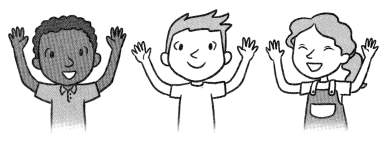6 × 5 = ____
They raise ____ fingers in all.
Explanation:
Given:
Number of children’s raise there hands = 6
Total number of fingers in each hand = 5
6 × 5 = 30
They raise 30 fingers in all.

Question 20.
Jeff buys 7 books at the bookstore. Each book costs $5. How much does Jeff pay for the 7 books?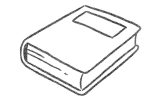He pays$_____ for the 7 books.
Each book cost = $5 To know the total amount Jeff pays for the book we need to multiply 7 with 5 so we get 7 × 5 = 35 He pays$35 for the 7 books.